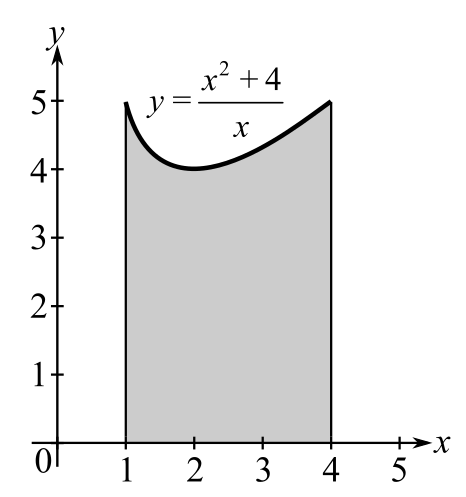Chapter 5.4, Problem 15E### Calculus: An Applied Approach (Min...

10th Edition
Ron Larson
ISBN: 9781305860919

#### Solutions

Chapter
Section### Calculus: An Applied Approach (Min...

10th Edition
Ron Larson
ISBN: 9781305860919
Textbook Problem
1 views

# Finding Area by the Fundamental Theorem In Exercises 9-16, find the area of the region. See Example 2. y = x 2 + 4 xTo determine

To calculate: The area of the region y=x2+4x in the provided figure.Explanation

Given Information:

The function is y=x2+4x and the provided figure is:

Formula used:

The fundamental theorem of calculus states that,

If f is integrable on interval [a,b] then abf(x)dx=F(b)F(a).

The integration formula is xndx=xn+1n+1+C and 1xdx=lnx+C

Calculation:

Consider the figure.

To find the area of the region, fundamental theorem of calculus can be used.

Integrate the function from x=1 to x=4 as shown below.

14(x2+4x)dx

This can be written as,

14(x2+4x

### Still sussing out bartleby?

Check out a sample textbook solution.

See a sample solution

#### The Solution to Your Study Problems

Bartleby provides explanations to thousands of textbook problems written by our experts, many with advanced degrees!

Get Started

#### Find more solutions based on key concepts